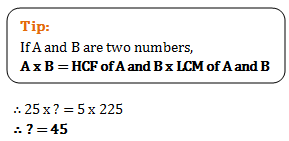# Problems on H.C.F and L.C.M - Quantitative Aptitude (MCQ) questions for Q. 29375

Q.  If least common multiple of two numbers is 225 and the highest common factor is 5 then find the numbers when one of the numbers is 25?
- Published on 11 Apr 17

a. 75
b. 65
c. 15
d. 45